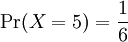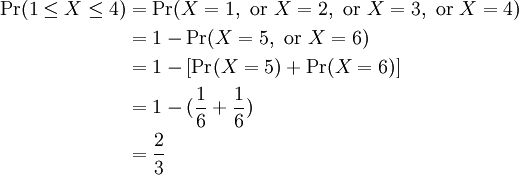# 1.2 – Notation

Previous: 1.1 – Random Variables

Next: 1.3 – The Discrete Probability Density Function

## Random Variables and their Observed Values

We commonly use uppercase letters to denote random variables, and lowercase letters to denote particular values that our random variables can assume.

For example, consider a six-sided die, pictured below.

We could let X be the random value that gives the value observed on the upper face of the six-sided die after a single roll. Then if x denotes a particular value of the upper face, the expression X = x becomes well-defined. Specifically, the notation X = x signifies the event that the random variable X assumes the particular value x. For the six-sided die example, x can be any integer from 1 to 6. So the expression X = 4 would express the event that a random roll of the die would result in observing the value 4 on the upper face of the die.

## Probabilities of Discrete Random Variables

We have already defined the notation Pr(X = x) to denote the probability that a random variable X is equal to a particular value x. Similarly, Pr(Xx) would denote the probability that the random variable X is less than or equal to the value x.

Notation
Pr(aXb) denotes the probability that the random variable X lies between values a and b, inclusively.

With this notation, it now makes sense to write, for example, Pr(X > a), the probability that a random variable assumes a particular value strictly greater than a. Similarly, we can make sense of the expressions Pr(X < b), Pr(Xx), Pr(X = x1 or X = x2), among others.

Notice that this notation allows us to do a kind of algebra with probabilities. For example, we notice the equivalence of the following two expressions: Pr(Xa and X < b) = Pr(aX < b). An important consequence of this symbolism is the following:

Probabilities of Complimentary Events
Pr(X = x) = 1 - Pr(Xx)
Pr(X > x) = 1 - Pr(Xx)
Pr(Xx) = 1 - Pr(X < x)

Notice that the first identity is simply a restatement of Discrete Probability Rule #3 from the previous page.

These three identities are simple consequences of our notation and of the fact that the sum of all probabilities must always equal 1 for any random variable. The events X = x and Xx are called complimentary because exactly one of the events must take place; i.e. both events cannot occur simultaneously, but one of the two must occur. The other expressions above also define complimentary events.

For discrete random variables, we also have the identity:

Algebra of Disjoint Events
If ab, then Pr(X = a or X = b) = Pr(X = a) + Pr(X = b)

## Six-Sided Die Example

Using our six-sided die example above, we have the random variable X which represents the value we observe on the upper face of the six-sided die after a single roll. Then the probability that X is equal to 5 can be written as:Using our identities for complimentary events and for disjoint events, we find that the probability that X is equal to 1, 2, 3 or 4 can be computed as:Notice that X ~ Uniform(6); i.e. X has a uniform distribution on the integers from 1 to 6. Indeed, the probability of observing any one of these integer values (the value on the upper face of the rolled die) is the same for any value. Thus, X must be a uniform random variable.

Previous: 1.1 – Random Variables

Next: 1.3 – The Discrete Probability Density Function